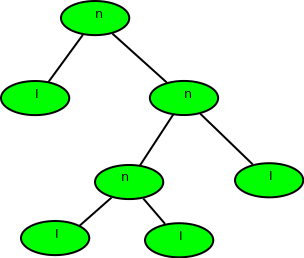Open in App
Not now

# Calculate depth of a full Binary tree from Preorder

• Difficulty Level : Medium
• Last Updated : 06 Jul, 2022

Given preorder of a binary tree, calculate its depth(or height) [starting from depth 0]. The preorder is given as a string with two possible characters.

1. ‘l’ denotes the leaf
2. ‘n’ denotes internal node

The given tree can be seen as a full binary tree where every node has 0 or two children. The two children of a node can ‘n’ or ‘l’ or mix of both.

Examples :

```Input  : nlnll
Output : 2
Explanation :``````Input  : nlnnlll
Output : 3```Preorder of the binary tree is given so traverse

Also, we would be given a string of char (formed of ‘n’ and ‘l’), so there is no need to implement tree also.

The recursion function would be:

1. Base Case: return 0; when tree[i] = ‘l’ or i >= strlen(tree)
2. find_depth( tree[i++] ) //left subtree
3. find_depth( tree[i++] ) //right subtree

Where i is the index of the string tree.

Implementation:

## C++

 `// C++ program to find height of full binary tree``// using preorder``#include ``using` `namespace` `std;` `// function to return max of left subtree height``// or right subtree height``int` `findDepthRec(``char` `tree[], ``int` `n, ``int``& index)``{``    ``if` `(index >= n || tree[index] == ``'l'``)``        ``return` `0;` `    ``// calc height of left subtree (In preorder``    ``// left subtree is processed before right)``    ``index++;``    ``int` `left = findDepthRec(tree, n, index);` `    ``// calc height of right subtree``    ``index++;``    ``int` `right = findDepthRec(tree, n, index);` `    ``return` `max(left, right) + 1;``}` `// Wrapper over findDepthRec()``int` `findDepth(``char` `tree[], ``int` `n)``{``    ``int` `index = 0;``    ``return` `findDepthRec(tree, n, index);``}` `// Driver program``int` `main()``{``    ``// Your C++ Code``    ``char` `tree[] = ``"nlnnlll"``;``    ``int` `n = ``strlen``(tree);` `    ``cout << findDepth(tree, n) << endl;` `    ``return` `0;``}`

## Java

 `// Java program to find height``// of full binary tree using``// preorder``import` `java .io.*;` `class` `GFG``{``    ``// function to return max``    ``// of left subtree height``    ``// or right subtree height``    ``static` `int` `findDepthRec(String tree,``                            ``int` `n, ``int` `index)``    ``{``        ``if` `(index >= n ||``            ``tree.charAt(index) == ``'l'``)``            ``return` `0``;` `        ``// calc height of left subtree``        ``// (In preorder left subtree``        ``// is processed before right)``        ``index++;``        ``int` `left = findDepthRec(tree,``                                ``n, index);` `        ``// calc height of``        ``// right subtree``        ``index++;``        ``int` `right = findDepthRec(tree, n, index);` `        ``return` `Math.max(left, right) + ``1``;``    ``}` `    ``// Wrapper over findDepthRec()``    ``static` `int` `findDepth(String tree,``                         ``int` `n)``    ``{``        ``int` `index = ``0``;``        ``return` `(findDepthRec(tree,``                             ``n, index));``    ``}` `    ``// Driver Code``    ``static` `public` `void` `main(String[] args)``    ``{``        ``String tree = ``"nlnnlll"``;``        ``int` `n = tree.length();``        ``System.out.println(findDepth(tree, n));``    ``}``}` `// This code is contributed``// by anuj_67.`

## Python3

 `#Python program to find height of full binary tree``# using preorder``    ` `# function to return max of left subtree height``# or right subtree height``def` `findDepthRec(tree, n, index) :` `    ``if` `(index[``0``] >``=` `n ``or` `tree[index[``0``]] ``=``=` `'l'``):``        ``return` `0` `    ``# calc height of left subtree (In preorder``    ``# left subtree is processed before right)``    ``index[``0``] ``+``=` `1``    ``left ``=` `findDepthRec(tree, n, index)` `    ``# calc height of right subtree``    ``index[``0``] ``+``=` `1``    ``right ``=` `findDepthRec(tree, n, index)``    ``return` `(``max``(left, right) ``+` `1``)` `# Wrapper over findDepthRec()``def` `findDepth(tree, n) :` `    ``index ``=` `[``0``]``    ``return` `findDepthRec(tree, n, index)` `        ` `# Driver program to test above functions``if` `__name__ ``=``=` `'__main__'``:``    ``tree``=` `"nlnnlll"``    ``n ``=` `len``(tree)` `    ``print``(findDepth(tree, n))` `# This code is contributed by SHUBHAMSINGH10`

## C#

 `// C# program to find height of``// full binary tree using preorder``using` `System;` `class` `GFG {` `    ``// function to return max of left subtree``    ``// height or right subtree height``    ``static` `int` `findDepthRec(``char``[] tree, ``int` `n, ``int` `index)``    ``{``        ``if` `(index >= n || tree[index] == ``'l'``)``            ``return` `0;` `        ``// calc height of left subtree (In preorder``        ``// left subtree is processed before right)``        ``index++;``        ``int` `left = findDepthRec(tree, n, index);` `        ``// calc height of right subtree``        ``index++;``        ``int` `right = findDepthRec(tree, n, index);` `        ``return` `Math.Max(left, right) + 1;``    ``}` `    ``// Wrapper over findDepthRec()``    ``static` `int` `findDepth(``char``[] tree, ``int` `n)``    ``{``        ``int` `index = 0;``        ``return` `(findDepthRec(tree, n, index));``    ``}` `    ``// Driver program``    ``static` `public` `void` `Main()``    ``{``        ``char``[] tree = ``"nlnnlll"``.ToCharArray();``        ``int` `n = tree.Length;``        ``Console.WriteLine(findDepth(tree, n));``    ``}``}` `// This code is contributed by vt_m.`

## Javascript

 ``

Output

`3`

Time Complexity: O(N)
Auxiliary Space: O(1)

This article is contributed by Shubham Gupta. If you like GeeksforGeeks and would like to contribute, you can also write an article using write.geeksforgeeks.org or mail your article to review-team@geeksforgeeks.org. See your article appearing on the GeeksforGeeks main page and help other Geeks.

My Personal Notes arrow_drop_up